# How To Enter A Fraction And Change The Format Of A Fraction In Excel

Feb 03, 2020 • edited Feb 04, 2020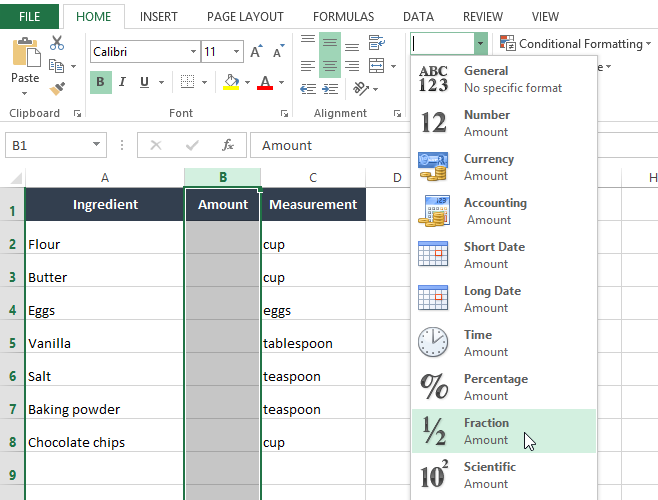If you've ever worked with Excel, chances are you've used it to store and calculate different types of values, such as whole numbers, decimals, and percentages. However, there may also be times when you want to work with fractions in Excel, such as 1/2 (one-half) or 2/3 (two-thirds), rather than use the decimal value.

This example teaches you how to enter a fraction in Excel and how change the format of a fraction.

1. To enter the mixed fraction 1 4/7 (one and four sevenths), type 1 4/7. To enter the fraction 4/7 (four sevenths) into cell A1, select cell A1 and type 0 4/7 (with a 0 or Excel thinks you want to enter a date).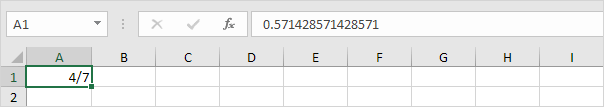Now that you've entered a fraction correctly, Excel has applied a Fraction format to cell A1 and you can edit the fraction by simply typing 5/7 (without a 0). You can find the decimal value of the fraction in the formula bar.

2. Let's take a look at the applied Fraction format. Select cell A1, right click, and then click Format Cells.

Excel has applied the Up to one digit type.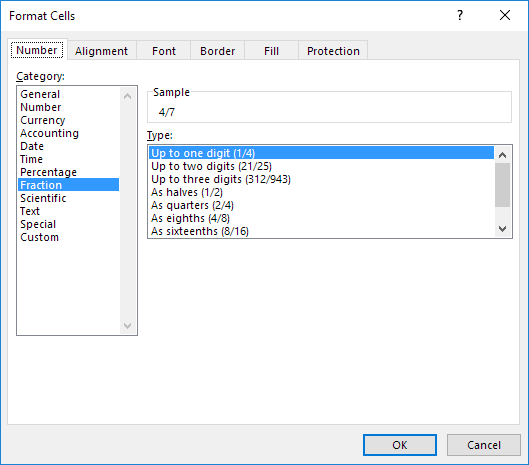Note: to enter a fraction like 4/11 into cell A1, change the type to Up to two digits. To enter a fraction like 1/148 into cell A1, change the type to Up to three digits.

3a. Excel rounds your fraction if it cannot display the right fraction. For example, if you type 4/11 into cell A1 (with the Up to one digit type applied), Excel displays 1/3. The formula bar always shows the correct decimal value.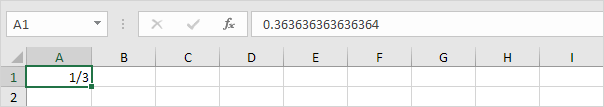3b. Change the type to Up to two digits.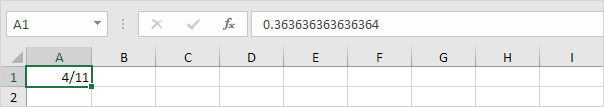Note: of course, if you type 0 4/11 into a new cell, Excel automatically applies the Up to two digits type and correctly displays 4/11.

4. Excel always reduces a fraction to its smallest denominator. If you enter the fraction 2/8, Excel converts the fraction to 1/4. However, you can use the As eights (4/8) type to fix the denominator to 8.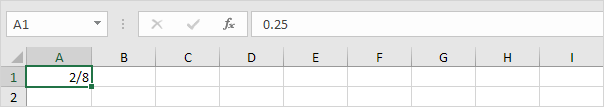5. Sometimes, you cannot find the right denominator for your fraction. For example, you want to display 40/50, but Excel converts 40/50 to 4/5. However, the As fiftieths (25/50) type is not available. Create a custom number format (# ??/50) to fix this.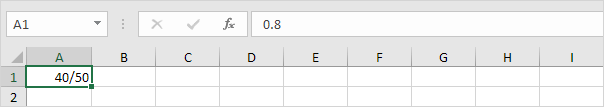Note: change the 50 to 60 to create the As sixtieths (30/60) type, etc.

#How To#Tutorial#Format Cells

The Currency and Accounting format in Excel

Dates and times displayed in a variety of ways in Excel